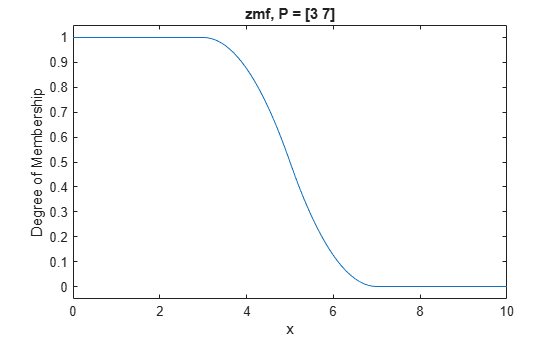zmf

Z-shaped membership function

Description

This function computes fuzzy membership values using a spline-based Z-shaped membership function. You can also compute this membership function using a fismf object. For more information, see fismf Object.

This membership function is related to the smf and pimf membership functions.

example

y = zmf(x,params) returns fuzzy membership values computed using the spline-based Z-shaped membership function given by:

$f\left(x;a,b\right)=\left\{\begin{array}{ll}1,\hfill & x\le a\hfill \\ 1-2{\left(\frac{x-a}{b-a}\right)}^{2},\hfill & a\le x\le \frac{a+b}{2}\hfill \\ \text{2}{\left(\frac{x-b}{b-a}\right)}^{\text{2}},\hfill & \frac{a+b}{2}\le x\le b\hfill \\ 0\hfill & x\ge b\hfill \end{array}$

To specify the a and b parameters, use params.

Membership values are computed for each input value in x.

Examples

collapse all

x = 0:0.1:10;
y = zmf(x,[3 7]);
plot(x,y)
xlabel('zmf, P = [3 7]')
ylim([-0.05 1.05])Input Arguments

collapse all

Input values for which to compute membership values, specified as a scalar or vector.

Membership function parameters, specified as the vector [a b]. Parameter a defines the shoulder of the membership function, and b defines its foot.

Output Arguments

collapse all

Membership value returned as a scalar or a vector. The dimensions of y match the dimensions of x. Each element of y is the membership value computed for the corresponding element of x.

Alternative Functionality

fismf Object

You can create and evaluate a fismf object that implements the zmf membership function.

mf = fismf("zmf",P);
Y = evalmf(mf,X);

Here, X, P, and Y correspond to the x, params, and y arguments of zmf, respectively.